# Math Tricks

📌 TIPS | HACKS WHICH YOU CAN'T IGNORE AS A CODER ✨🎩 - LeetCode Discuss

Whenever you get an integer conversion problem, think of modulo % and floor division //

6.2 epi

• Reverse Integer

"""
Reverse Integer:

Given a 32-bit signed integer, reverse digits of an integer.
Assume we are dealing with an environment that could only store integers within the 32-bit signed integer range: [−231,  231 − 1].
For the purpose of this problem, assume that your function returns 0 when the reversed integer overflows.

https://leetcode.com/problems/reverse-integer/
"""

class Solution:
def reverse(self, x):
res = 0

num = abs(x)
while num > 0:
last_digit = num % 10 # get last digit
res = (10 * res) + last_digit

num //= 10  # remove last digit

# confirm 32-bit signed integer
if res < -2**31 or res > 2**31-1:
return 0

#
if x < 0:
return -res
return res

class SolutionB:
def reverse(self, x: int):

# check if negative
negative = False
if x < 0:
negative = True

num = list(str(x))
rev_num = []

# skip the minus sign(for negative values)
length = len(num)
maximum = length
if negative:
maximum = length - 1

# reverse each character
i = 0
while i < maximum:
rev_num.append(num.pop())
i += 1

# create new integer
res = int("".join(rev_num))
if negative:
res = -res

# confirm 32-bit signed integer
if res < -2**31 or res > 2**31-1:
return 0
return res

• Valid Number

"""
Valid Number

A valid number can be split up into these components (in order):
A decimal number or an integer.
(Optional) An 'e' or 'E', followed by an integer.
A decimal number can be split up into these components (in order):
(Optional) A sign character (either '+' or '-').
One of the following formats:
One or more digits, followed by a dot '.'.
One or more digits, followed by a dot '.', followed by one or more digits.
A dot '.', followed by one or more digits.
An integer can be split up into these components (in order):
(Optional) A sign character (either '+' or '-').
One or more digits.

For example,
all the following are valid numbers:
["2", "0089", "-0.1", "+3.14", "4.", "-.9", "2e10", "-90E3", "3e+7", "+6e-1", "53.5e93", "-123.456e789"],
while the following are not valid numbers:
["abc", "1a", "1e", "e3", "99e2.5", "--6", "-+3", "95a54e53"].
Given a string s, return true if s is a valid number.

Example 1:
Input: s = "0"
Output: true
Example 2:
Input: s = "e"
Output: false
Example 3:
Input: s = "."
Output: false
Example 4:
Input: s = ".1"
Output: true

https://leetcode.com/problems/valid-number
"""

from collections import Counter

# O(N) time | O(N) space
class Solution:
def isNumber(self, s: str):
"""
- verify all characters are 0-9,e,E,+,-,.
- if has e/E's:
- run the verifyNumberWithEs(s)
- else:
- run the verifyNumberWithoutEs(s)
"""
if not s:
return False
# verify all characters are 0-9,e,E,+,-,.
for char in s:
if not (char.isnumeric() or char in "eE+-."):
return False
# if has e/E's
if 'e' in s or 'E' in s:
return self.verifyNumberWithEs(s)
else:
return self.verifyNumberWithoutEs(s)

def verifyNumberWithEs(self, s):
"""
- verify e/E's
- only one

- split s by the E's
- followed by integer (not float)
- order: [number, e, number]
- run the verifyNumberWithoutEs() on the numbers
"""

# only one E
char_count = Counter(s)
if char_count['e'] + char_count['E'] != 1:
return False

# split s
e_split = []
if 'e' in char_count:
e_split = s.split("e")
else:
e_split = s.split("E")
if len(e_split) != 2:
return False

# followed by integer (not float)
if "." in e_split:
return False

# run the verifyNumberWithoutEs() on the numbers
return self.verifyNumberWithoutEs(e_split) and self.verifyNumberWithoutEs(e_split)

def verifyNumberWithoutEs(self, s):
"""
Checks if a number is valid without considering e/E's
- has numeric characters
- check if has zero or one of -,+ at the beginning and remove it
- check if has zero or one of .
- verify . has digits on either side
"""
char_count = Counter(s)

# has numeric characters
if not self.has_numeric_chars(s):
return False

# check if has zero or one of -,+ at the beginning and remove it
sign_count = char_count['+'] + char_count['-']
if not (sign_count == 1 or sign_count == 0):
return False
s_wo_signs = s
if sign_count:
s_wo_signs = s[1:]
if not self.has_numeric_chars(s_wo_signs):
return False
if '+' in s_wo_signs or '-' in s_wo_signs:
return False

# check if has zero or one of .
if not (char_count['.'] == 1 or char_count['.'] == 0):
return False
# verify . has digits on either side
dot_split = s_wo_signs.split('.')
if not self.has_numeric_chars(dot_split) and not self.has_numeric_chars(dot_split):
return False

return True

def has_numeric_chars(self, s):
for char in s:
if char.isnumeric():
return True
return False

"""
Constant space

"""

# O(N) time | O(1) space
class Solution_:
def isNumber(self, s: str):
"""
Digits
- must exist
Signs
- one on either side of exponent
- at beginning of string or just after exponent
- must have digits after it
Exponents
- must have digits b4 & after it
- can only be one
Dots
- cannot be after exponent
- can be only on left side of exponent
- max of one
- digit on either side
Anything else
- invalid input
"""

seen_digit = False
seen_exponent = False
seen_dot = False
for idx, char in enumerate(s):
# 0-9
if char.isnumeric():
seen_digit = True

# "+-" one on either side of exponent
# can be at beginning of string or just after exponent & must have digits after it
elif char in "+-":
if not (idx == 0 or s[idx-1] in "eE") or idx == len(s)-1:
return False

# "eE" - must have digits b4 & after it & can only be one
elif char in "eE":
if not seen_digit or seen_exponent or idx == len(s)-1:
return False
seen_exponent = True

# "." max of one & can be only on left side of exponent & digit on either side
elif char == ".":
# max of one & can be only on left side of exponent
if seen_dot or seen_exponent:
return False
# digit on either side
has_left_digit = False
has_right_digit = False
if idx > 0 and s[idx-1].isnumeric():
has_left_digit = True
if idx < len(s)-1 and s[idx+1].isnumeric():
has_right_digit = True

if not (has_left_digit or has_right_digit):
return False
seen_dot = True

# invalid character
else:
return False

# digits must exist
return seen_digit

"""
test cases:
"2"
"0089"
"-0.1"
"+3.14"
"4."
"-.9"
"2e10"
"-90E3"
"3e+7"
"+6e-1"
"53.5e93"
"-123.456e789"
"abc"
"1a"
"1e"
"e3"
"99e2.5"
"--6"
"-+3"
"95a54e53"
"""

• Maximum Swap

"""
670. Maximum Swap:

You are given an integer num. You can swap two digits at most once to get the maximum valued number.
Return the maximum valued number you can get.

Example 1:
Input: num = 2736
Output: 7236
Explanation: Swap the number 2 and the number 7.
Example 2:
Input: num = 9973
Output: 9973
Explanation: No swap.

Needs many examples to understand
Drawing a chart of heaps and valleys can make it a bit easier to understand

4123 => 4321
4312 => 4321
4321 => 4321
98368 => 98863
1993 => 9913

https://leetcode.com/problems/maximum-swap

similar to https://leetcode.com/problems/next-permutation/
"""

# use two pointers
class Solution_:
def maximumSwap(self, num: int):
"""
Ensure the largest value is as left as possible
"""
num_arr = list(str(num))

for i in range(len(num_arr)):
# find largest that is as right as possible: eg 1993 => 9913
largest = i
for idx in range(i+1, len(num_arr)):
if int(num_arr[idx]) >= int(num_arr[largest]):
largest = idx

# if we found a larger value, swap & return
if int(num_arr[largest]) > int(num_arr[i]):
num_arr[largest], num_arr[i] = num_arr[i], num_arr[largest]
return int("".join(num_arr))

return num

"""

"""

class Solution:
def maximumSwap(self, num: int):
"""
Ensure the largest values are as left as possible
"""
num_arr = list(str(num))
largest_idx_arr = list(range(len(num_arr)))

# fill in the largest values to the right for each index in the array
largest = len(num_arr)-1
for idx in reversed(range(len(num_arr))):
if int(num_arr[idx]) > int(num_arr[largest]):
largest = idx
largest_idx_arr[idx] = largest

# swap left most
for idx in range(len(num_arr)):
largest_idx = largest_idx_arr[idx]
# ignore same size numbers
if num_arr[idx] == num_arr[largest_idx]:
continue

# swap and return
num_arr[largest_idx], num_arr[idx] = num_arr[idx], num_arr[largest_idx]
return int("".join(num_arr))

return num

• Next Permutation **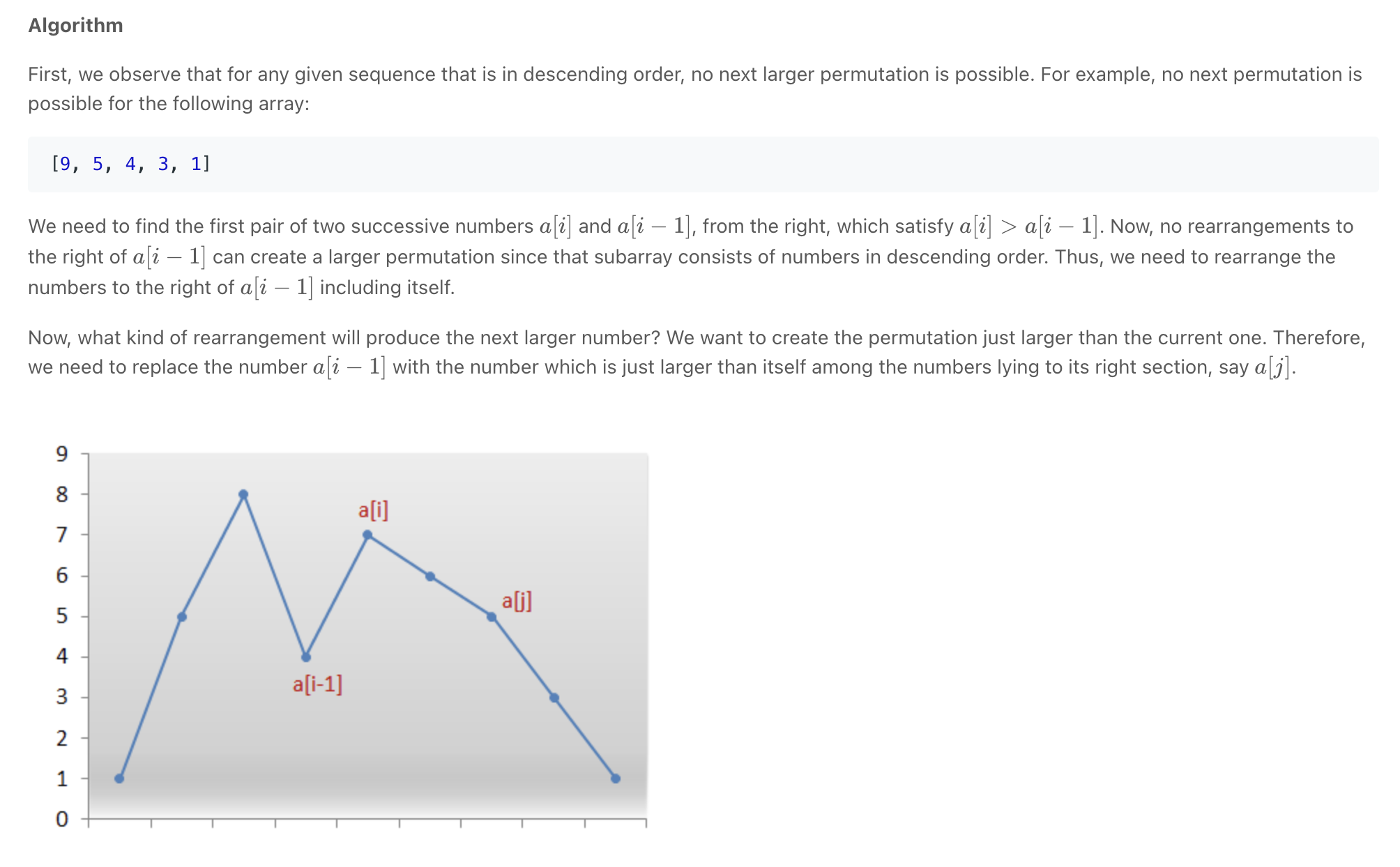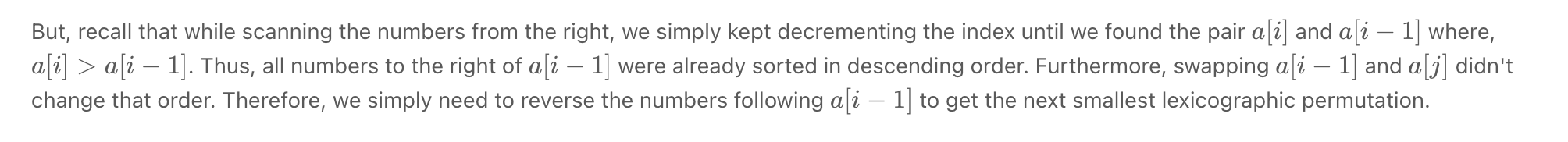"""
Next Permutation:

Implement next permutation, which rearranges numbers into the lexicographically next greater permutation of numbers.
If such an arrangement is not possible,
it must rearrange it as the lowest possible order (i.e., sorted in ascending order).
The replacement must be in place and use only constant extra memory.

Example 1:
Input: nums = [1,2,3]
Output: [1,3,2]
Example 2:
Input: nums = [3,2,1]
Output: [1,2,3]
Example 3:
Input: nums = [1,1,5]
Output: [1,5,1]
Example 4:
Input: nums = 
Output: 

Needs many examples to understand
Drawing a chart of heaps and valleys can make it a bit easier to understand

[1,3,2] => [2,1,3]

[1,2,3,4] => [1,2,4,3]
[4,1,2,3] => [4,1,3,2]
[3,4,2,1] => [4,1,2,3] - swapped 3 & 4 then sorted the numbers to the right of 4
[2,3,4,1] => [2,4,1,3] - swapped 3 & 4 then sorted the numbers to the right of 4

[15,2,--4--,6,--5--,2] => [15,2,--5--,2,4,6] - swapped 4 & 5 then sorted the numbers to the right of 5

https://leetcode.com/problems/next-permutation/
https://www.notion.so/paulonteri/Math-Tricks-8c99fd21a1d343f7bee1eaf0467ea362#4bda6bec59634b1ebf5bc34fb2edc542

similar to https://leetcode.com/problems/maximum-swap
"""

class Solution:
def nextPermutation(self, nums):
"""
1. look for peak that has its larger & smaller number furthest to the right
- Note: peak => num[right] > num[left]
- find **first smaller number** (furthest to the right)
- then **find the number furthest to the right that is larger than it**
- swap the smaller and larger
2. if no peak was found srt the whole array and return
3. sort the array to the right of where the larger number was placed

"""
small_num_idx = None

# find first smaller number (furthest to the right)
i = len(nums)-1
largest = nums[-1]
while not small_num_idx and i >= 0:
if nums[i] < largest:
small_num_idx = i
largest = max(largest, nums[i])
i -= 1

# array is sorted in descending order
if small_num_idx is None:
nums.sort()
return

# find number (furthest to the right) larger than small_num
for idx in reversed(range(small_num_idx+1, len(nums))):
if nums[idx] > nums[small_num_idx]:
# swap larger and smaller number
nums[small_num_idx], nums[idx] = nums[idx], nums[small_num_idx]
# sort area after where the larger swapped number was placed
self.sort(nums, small_num_idx+1)

return

def sort(self, nums, sort_start):
# nums[sort_start:] = list(sorted(nums[sort_start:]))
for idx in range(sort_start, len(nums)):
smallest = idx
for i in range(idx, len(nums)):
if nums[i] < nums[smallest]:
smallest = i
nums[smallest], nums[idx] = nums[idx], nums[smallest]

• String to Integer & Integer to String

"""
Integer to String
"""

def single_digit_to_char(digit):
return chr(ord('0')+digit)

def int_to_string(x: int):
if x == 0:
return "0"

is_neg = False
if x < 0:
is_neg, x = True, -x

result = []
while x > 0:
digit = x % 10
result.append(single_digit_to_char(digit))
x = x // 10

if is_neg:
result.append('-')
return "".join(reversed(result))

"""
String to Integer
"""

def single_char_to_int(character):
# num_mapping = {
#     "1": 1,
#     "2": 2,
#     "3": 3,
#     "4": 4,
#     "5": 5,
#     "6": 6,
#     "7": 7,
#     "8": 8,
#     "9": 9,
#     "0": 0,
# }
# return num_mapping[character]
return ord(character) - ord('0')

def string_to_int(s: str):
is_neg = False
num = 0
multiplier = 1

start_idx = 0
if s and s == "-":
is_neg, start_idx = True, 1
if s and s == "+":
start_idx = 1
for idx in reversed(range(start_idx, len(s))):
num += single_char_to_int(s[idx])*multiplier
multiplier *= 10

if is_neg:
return -num
return num

print(string_to_int("22"), type(string_to_int("22")))

• String to Integer (atoi)

"""
String to Integer (atoi):

Implement atoi which converts a string to an integer.
The function first discards as many whitespace characters as necessary until the first non-whitespace character is found.
Then, starting from this character takes an optional initial plus or minus sign followed by as many numerical digits as possible, and interprets them as a numerical value.
The string can contain additional characters after those that form the integral number, which are ignored and have no effect on the behavior of this function.
If the first sequence of non-whitespace characters in str is not a valid integral number,
or if no such sequence exists because either str is empty or it contains only whitespace characters, no conversion is performed.
If no valid conversion could be performed, a zero value is returned.

Only the space character ' ' is considered a whitespace character.
Assume we are dealing with an environment that could only store integers within the 32-bit signed integer range: [−231,  231 − 1]. If the numerical value is out of the range of representable values, 231 − 1 or −231 is returned.
https://leetcode.com/problems/string-to-integer-atoi/
"""

class Solution:
def myAtoi(self, string: str):

idx = 0

# skip whitespace
while idx < len(string) and string[idx] == ' ':
idx += 1

# deal with number
if idx < len(string) and (string[idx] == '-' or string[idx] == '+' or string[idx].isnumeric()):
# find number
start = idx
if string[idx] == '-' or string[idx] == '+':  # handle stuff like "+-12"
idx += 1
while idx < len(string) and string[idx].isnumeric():
idx += 1

# convert into number
if string[start] == '-':
if idx-start == 1:
return 0
return -self.convertToIntNeg(string[start+1:idx])
elif string[start] == '+':
if idx-start == 1:
return 0
return self.convertToIntPos(string[start+1:idx])
return self.convertToIntPos(string[start:idx])

return 0

def convertToIntPos(self, string):
integer = int(string)
if integer >= 2**31:
return 2**31 - 1
return integer

def convertToIntNeg(self, string):
integer = int(string)
if integer >= 2**31:
return 2**31
return integer

"""
# Input: valid string
# Output: integer
# Assumptions:
- only ' ' is considered whitespace
- some strings will not contain numerical digits after the whitespace
- ignore everythong after the whitespace thet is not a -, + , or a numerical value
- return 0 if no valis sol is found

# Examples:
'  -502apple' -> -502
'  -502 apple' -> -502
'  -502 200' -> -502
'+502 200' -> 502
'  t-502apple' -> 0

# # First Approach:
- iterate through the string
# skip all the whitespace (while loop 1)
# check whether next character is -, +  or number: (while loop 2)
- if not, return 0
- if it is: continue iterating while storing all it's characters in an array ### O(1) time
# convert the stored characters into a number and return the number ### O(n) time
- convert the array into an string (skipping -, +) then return it or negate and return it
# return 0
## time O(n) | O(n) space - where n is len(string)

# # Second Approach:
- iterate through the string
# skip all the whitespace (while loop 1)
# check whether next character is -, +  or number: (while loop 2)
- if not, return 0
- if it is: continue iterating (keep track of the satrting and ending indices) ### O(1) time
# convert the found number string (we can get it using slicing) into a number and return the number ### O(n) time
- return it or negate and return it
# return 0
## time O(n) | O(n) space - where n is len(string)

def myAtoi(self, s: str):
idx = 0

# skip whitespace
while idx < len(string) and string[idx] == ' ':
idx += 1

# deal with number
if idx < len(string) and (string[idx] == '-' or string[idx] == '+' or string[idx].isnumeric())
# find number
start = idx
while idx < len(string) and (string[idx] == '-' or string[idx] == '+' or string[idx].isnumeric())
idx += 1

# convert into number
if string[start] == '-':
return -int(string[start+1:idx])
elif string[start] == '-':
return int(string[start+1:idx])
return int(string[start:idx])

return 0

"""

• Multiply Strings *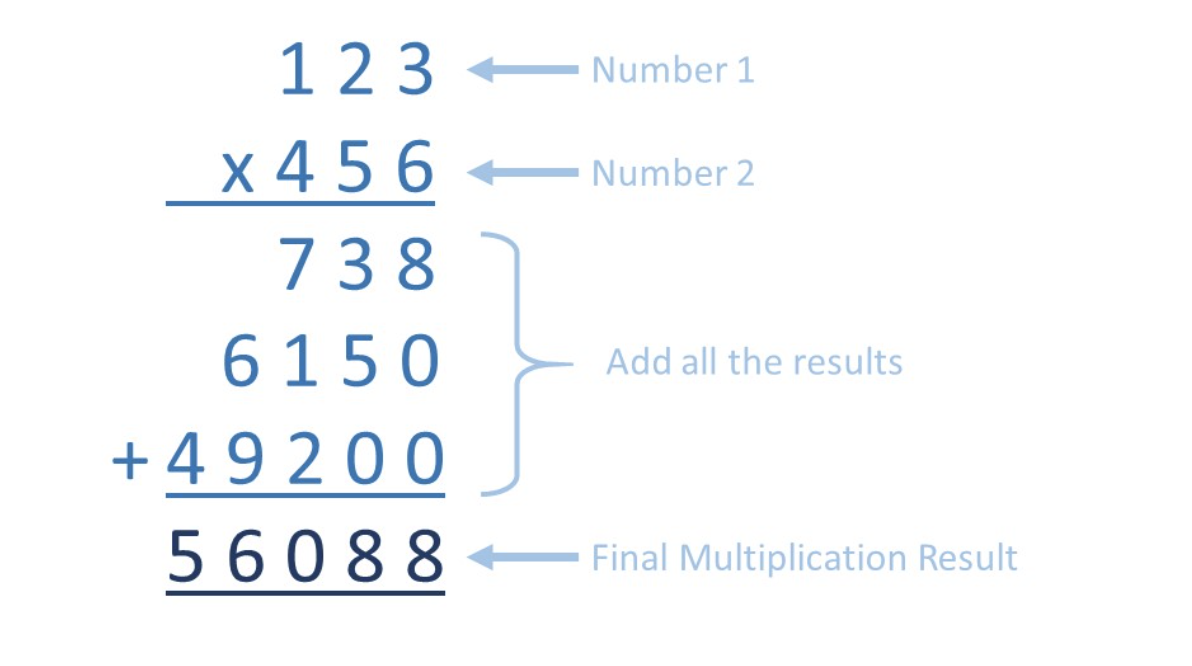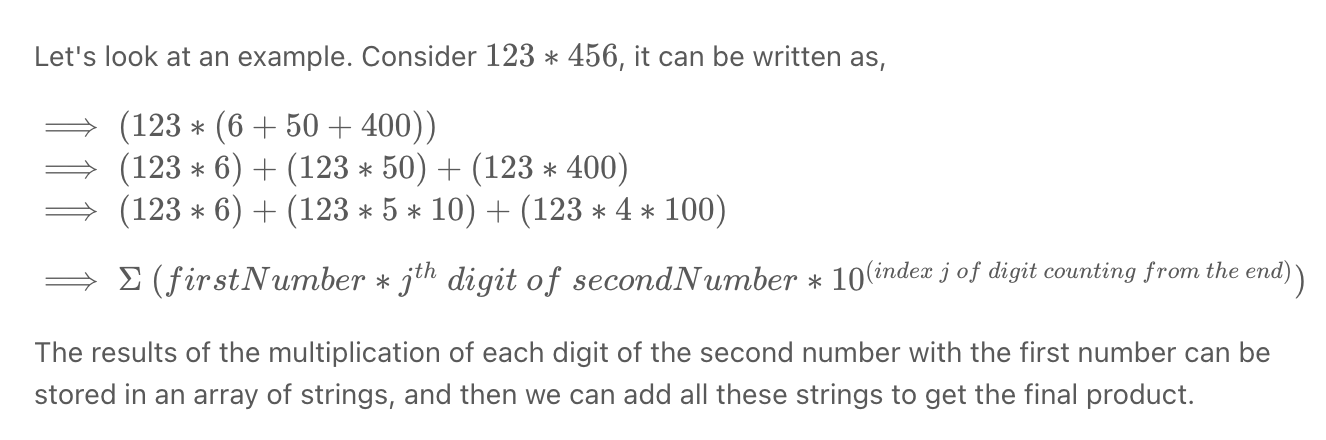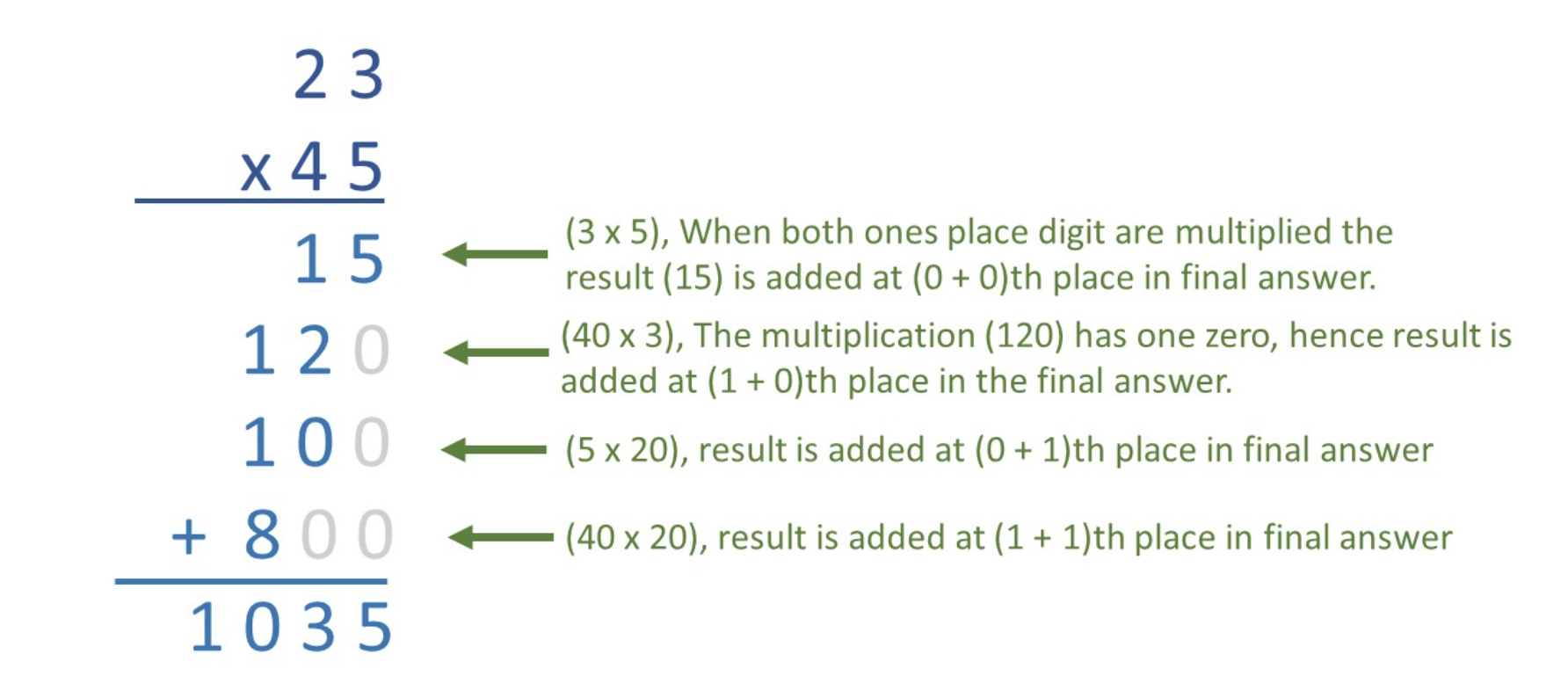"""
Multiply Strings:

Given two non-negative integers num1 and num2 represented as strings,
return the product of num1 and num2, also represented as a string.

Note: You must not use any built-in BigInteger library or convert the inputs to integer directly.

Example 1:
Input: num1 = "2", num2 = "3"
Output: "6"
Example 2:
Input: num1 = "123", num2 = "456"
Output: "56088"

https://leetcode.com/problems/multiply-strings
"""

class Solution:
def multiply(self, num1: str, num2: str):
if num1 == "0" or num2 == "0":
return "0"
res =  * (len(num1) + len(num2))

for i_one in reversed(range(len(num1))):
for i_two in reversed(range(len(num2))):
# +1 is used to handle (0,0) i_one=0 i_two=0 carries
# placing their carry will be easier if we move every element one step back/ to the right
pos = i_one + i_two + 1

carry = res[pos]
multiplication = (int(num1[i_one]) * int(num2[i_two])) + carry

# save
res[pos] = multiplication % 10
# place carry
res[pos-1] += multiplication // 10

idx = 0
while res[idx] == 0:
idx += 1
res = res[idx:]

return "".join([str(num) for num in res])

• Pascal's Triangle

"""
Pascal's Triangle

Given an integer numRows, return the first numRows of Pascal's triangle.

Example 1:
Input: numRows = 5
Output: [,[1,1],[1,2,1],[1,3,3,1],[1,4,6,4,1]]
Example 2:
Input: numRows = 1
Output: []

https://leetcode.com/problems/pascals-triangle
"""

class Solution:
def generate(self, numRows: int):
triangle = []
if numRows < 1:
return triangle

triangle.append()

row = []

# we handle the first and last indices separately
row.append(1)  # first index

# items to calculate/add will always be equal to the index (i) - 1
# for example in the 4th row (i=3) we calculate 2 values
for x in range(1, i):
# we know that each character is the sum of those above it: above left and above right
row.append(triangle[i-1][x-1] + triangle[i-1][x])

row.append(1)  # last index for row

triangle.append(row)

return triangle

• Roman to Integer

"""
Roman to Integer

Roman numerals are represented by seven different symbols: I, V, X, L, C, D and M.
Symbol       Value
I             1
V             5
X             10
L             50
C             100
D             500
M             1000
For example, 2 is written as II in Roman numeral, just two one's added together.
12 is written as XII, which is simply X + II. The number 27 is written as XXVII, which is XX + V + II.

Roman numerals are usually written largest to smallest from left to right.
However, the numeral for four is not IIII. Instead, the number four is written as IV.
Because the one is before the five we subtract it making four. The same principle applies to the number nine, which is written as IX.
There are six instances where subtraction is used:
I can be placed before V (5) and X (10) to make 4 and 9.
X can be placed before L (50) and C (100) to make 40 and 90.
C can be placed before D (500) and M (1000) to make 400 and 900.
Given a roman numeral, convert it to an integer.

Example 1:
Input: s = "III"
Output: 3
Example 2:
Input: s = "IV"
Output: 4
Example 3:
Input: s = "IX"
Output: 9
Example 4:
Input: s = "LVIII"
Output: 58
Explanation: L = 50, V= 5, III = 3.
Example 5:
Input: s = "MCMXCIV"
Output: 1994
Explanation: M = 1000, CM = 900, XC = 90 and IV = 4.

https://leetcode.com/problems/roman-to-integer
"""

class Solution_:
def romanToInt(self, s: str):

key_map = {
"I": 1,
"V": 5,
"X": 10,
"L": 50,
"C": 100,
"D": 500,
"M": 1000
}

num = 0

idx = 0
while idx < len(s):
if s[idx] == "I":
if idx+1 < len(s) and s[idx+1] == "V":
num += 4
idx += 2
elif idx+1 < len(s) and s[idx+1] == "X":
num += 9
idx += 2
else:
num += key_map[s[idx]]
idx += 1

elif s[idx] == "X":
if idx+1 < len(s) and s[idx+1] == "L":
num += 40
idx += 2
elif idx+1 < len(s) and s[idx+1] == "C":
num += 90
idx += 2
else:
num += key_map[s[idx]]
idx += 1

elif s[idx] == "C":
if idx+1 < len(s) and s[idx+1] == "D":
num += 400
idx += 2
elif idx+1 < len(s) and s[idx+1] == "M":
num += 900
idx += 2
else:
num += key_map[s[idx]]
idx += 1

else:
num += key_map[s[idx]]
idx += 1
return num

"""

"""

class Solution:
def romanToInt(self, s: str):
key_map = {
"I": 1,
"V": 5,
"X": 10,
"L": 50,
"C": 100,
"D": 500,
"M": 1000
}

num = 0
idx = 0
while idx < len(s):

if idx+1 < len(s) and key_map[s[idx]] < key_map[s[idx+1]]:
num += key_map[s[idx+1]] - key_map[s[idx]]
idx += 2
else:
num += key_map[s[idx]]
idx += 1

return num

• Basic Calculator II

"""
Basic Calculator II:

Given a string s which represents an expression, evaluate this expression and return its value.
The integer division should truncate toward zero.
You may assume that the given expression is always valid. All intermediate results will be in the range of [-231, 231 - 1].
Note: You are not allowed to use any built-in function which evaluates strings as mathematical expressions, such as eval().

Example 1:
Input: s = "3+2*2"
Output: 7
Example 2:
Input: s = " 3/2 "
Output: 1
Example 3:
Input: s = " 3+5 / 2 "
Output: 5

https://leetcode.com/problems/basic-calculator-ii/
"""

import math
"""
-------------------------- PROBLEM ----------------------------------
string s which represents an expression, evaluate this expression and return its value
not allowed to use any built-in function which evaluates strings as mathematical expressions
integer division should truncate toward zero.
s represents a valid expression
s consists of integers and operators ('+', '-', '*', '/')

-------------------------- EXAMPLES ----------------------------------
D/MA/S

"1+2"   => 3
"3+2*2" => 3+4 = 7
"5/2+3" => 2+3 = 5
"3+5/2" => 3+2 = 5
"3+5/2+5/2" => 3+2+2 = 7

-------------------------- BRUTE FORCE ----------------------------------
O(n^2) time | O(1) time
-  evaluate each of DMAS and add it back to the string
- do division, add it back to the string
- multiplication...

-------------------------- OPTIMAL ----------------------------------

-------------------------- ONE
O(n) time | O(1) time

- separate the s into an array that contain intergers and the signs

-  evaluate each of D/MA/S and add it to an array
- do division on array, add the results to after_div array
- do multiplication on after_div, add the results to after_mult array

-------------------------- TWO:

stack = 
current_number = ""
prev_operand = "+"

# deal with * and /:
- iterate through the array:
- try to build up a number while the characters are numeric
- if you get to a sign:
- if the prev_operand is * or / :
- get the currentNumber and the prevNumber and apply the prev_operand on them then add the result to the stack
- if the prev_operand is -:
- add neg the number to the stack
- if the prev_operand is +:
- add the number to the stack

- record the new prev_operand
- reset current_number = ""
- add all the number in the stack

"""

"""
------------------------------------------------------------------------------------------
"""

class Solution1:
def calculate(self, s: str):

# separate the s into an array that contain integers and the signs
arr = []
i = 0
while i < len(s):
if s[i].isnumeric():
end = i
while end+1 < len(s) and s[end+1].isnumeric():
end += 1
arr.append(int(s[i:end+1]))
i = end + 1
elif s[i] == " ":
i += 1
else:
arr.append(s[i])
i += 1

# division or multiplication
after_dm = []
i = 0
while i < len(arr):
# check for division
if arr[i] == "/":
after_dm[-1] = after_dm[-1] // arr[i+1]
i += 2
# check for multiplication
elif arr[i] == "*":
after_dm[-1] = after_dm[-1] * arr[i+1]
i += 2
else:
after_dm.append(arr[i])
i += 1

after_sa = []
i = 0
while i < len(after_dm):
# check for subtraction
if after_dm[i] == "-":
after_sa[-1] = after_sa[-1] - after_dm[i+1]
i += 2
elif after_dm[i] == "+":
after_sa[-1] = after_sa[-1] + after_dm[i+1]
i += 2
else:
after_sa.append(after_dm[i])
i += 1

return "".join([str(item) for item in after_sa])

"""
------------------------------------------------------------------------------------------
"""

class Solution2:
def calculate(self, s: str):

# separate the s into an array that contain integers and the signs
arr = []
i = 0
while i < len(s):
if s[i].isnumeric():
end = i
while end+1 < len(s) and s[end+1].isnumeric():
end += 1
arr.append(s[i:end+1])
i = end + 1
elif s[i] == " ":
i += 1
else:
arr.append(s[i])
i += 1

stack = []
current_number = ""
prev_operand = "+"

for idx in range(len(arr)+1):
if idx < len(arr) and arr[idx].isnumeric():
current_number = arr[idx]
continue

if prev_operand == "-":
stack.append(-int(current_number))

elif prev_operand == "+":
stack.append(int(current_number))

elif prev_operand == "*":
prev_num = stack.pop()
stack.append(prev_num * int(current_number))

elif prev_operand == "/":
prev_num = stack.pop()
stack.append(math.trunc(prev_num / int(current_number)))

if idx < len(arr):
prev_operand = arr[idx]

number = 0
while stack:
number += stack.pop()

return number

"""

"""

class Solution3:
def calculate(self, s: str):

# separate the s into an array that contain integers and the signs
arr = []
i = 0
while i < len(s):
if s[i].isnumeric():
end = i
while end+1 < len(s) and s[end+1].isnumeric():
end += 1
arr.append(s[i:end+1])
i = end + 1
elif s[i] == " ":
i += 1
else:
arr.append(s[i])
i += 1

stack = []
for idx in range(len(arr)):
if not arr[idx].isnumeric():
continue

current_number = arr[idx]
# ignore first number
if idx == 0:
stack.append(int(current_number))
continue

prev_operand = arr[idx-1]

if prev_operand == "-":
stack.append(-int(current_number))

elif prev_operand == "+":
stack.append(int(current_number))

elif prev_operand == "*":
prev_num = stack.pop()
stack.append(prev_num * int(current_number))

elif prev_operand == "/":
prev_num = stack.pop()
stack.append(math.trunc(prev_num / int(current_number)))

if idx < len(arr):
prev_operand = arr[idx]

number = 0
while stack:
number += stack.pop()

return number

• 'K' Closest Points to the Origin

• Angle Between Hands of a Clock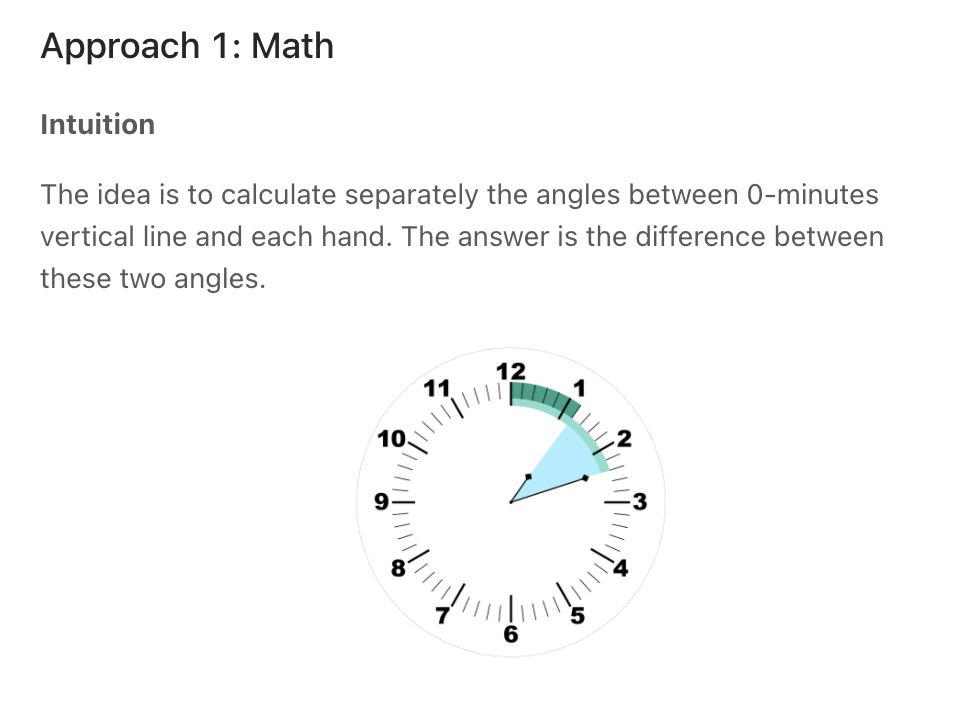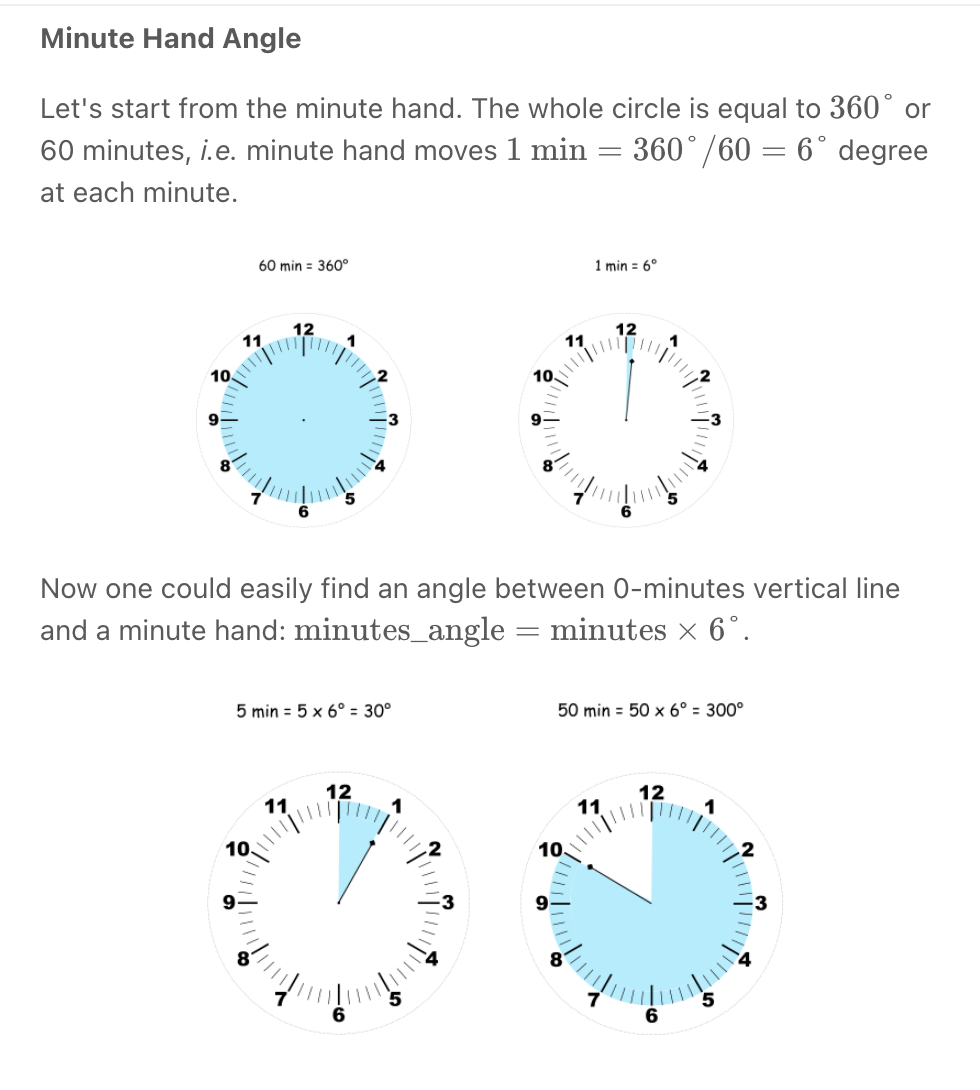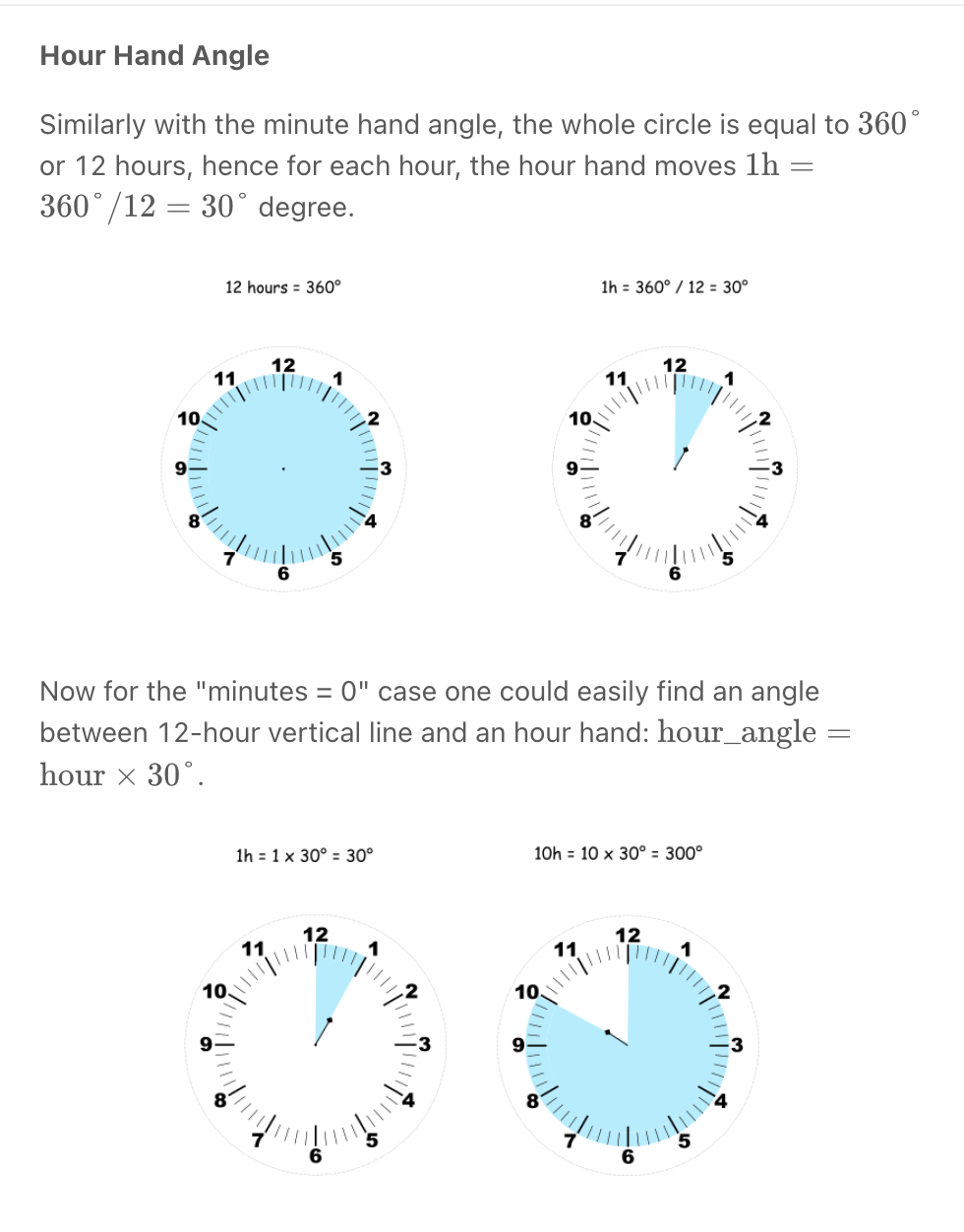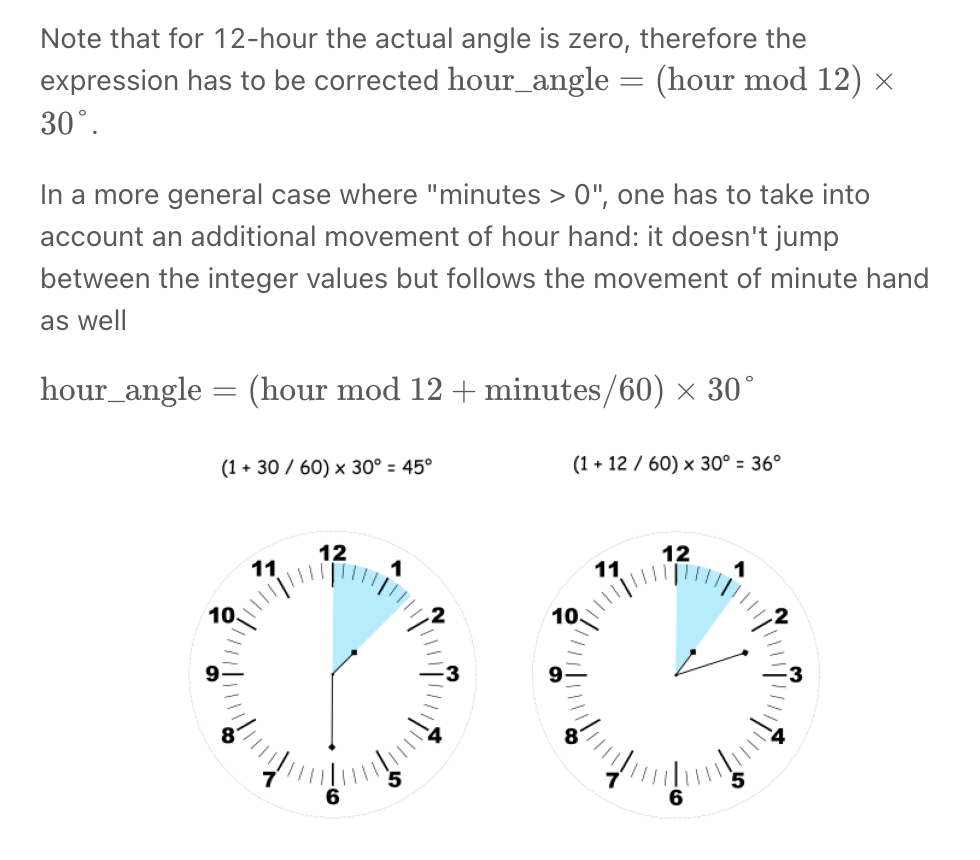"""
Angle Between Hands of a Clock

Given two numbers, hour and minutes.
Return the smaller angle (in degrees) formed between the hour and the minute hand.

Example 1:
Input: hour = 12, minutes = 30
Output: 165
Example 2:
Input: hour = 3, minutes = 30
Output: 75
Example 3:
Input: hour = 3, minutes = 15
Output: 7.5
Example 4:
Input: hour = 4, minutes = 50
Output: 155
Example 5:
Input: hour = 12, minutes = 0
Output: 0

https://leetcode.com/problems/angle-between-hands-of-a-clock
"""

class Solution:
def angleClock(self, hour: int, minutes: int):
"""
- calculate hour degree
(hour/12 * 360) + (minute/60 * 30)
- calculate minute degree
- minute/60 * 360
"""
hours = hour
if hour == 12:
hours = 0

hours_degree = (360 * hours/12) + (30 * minutes/60)
minutes_degree = 360 * minutes/60

diff = abs(hours_degree-minutes_degree)
return min(diff, abs(360-diff))

• Count Primes *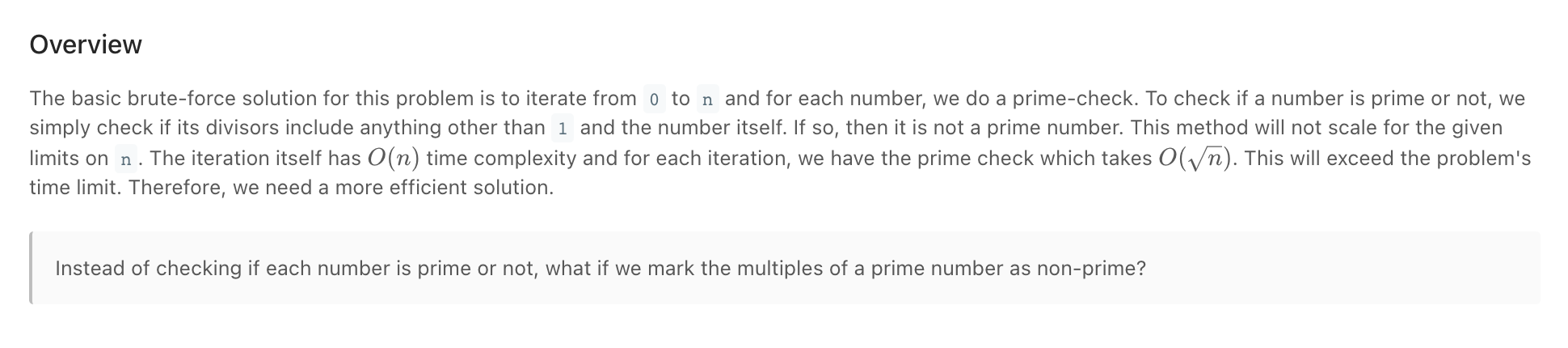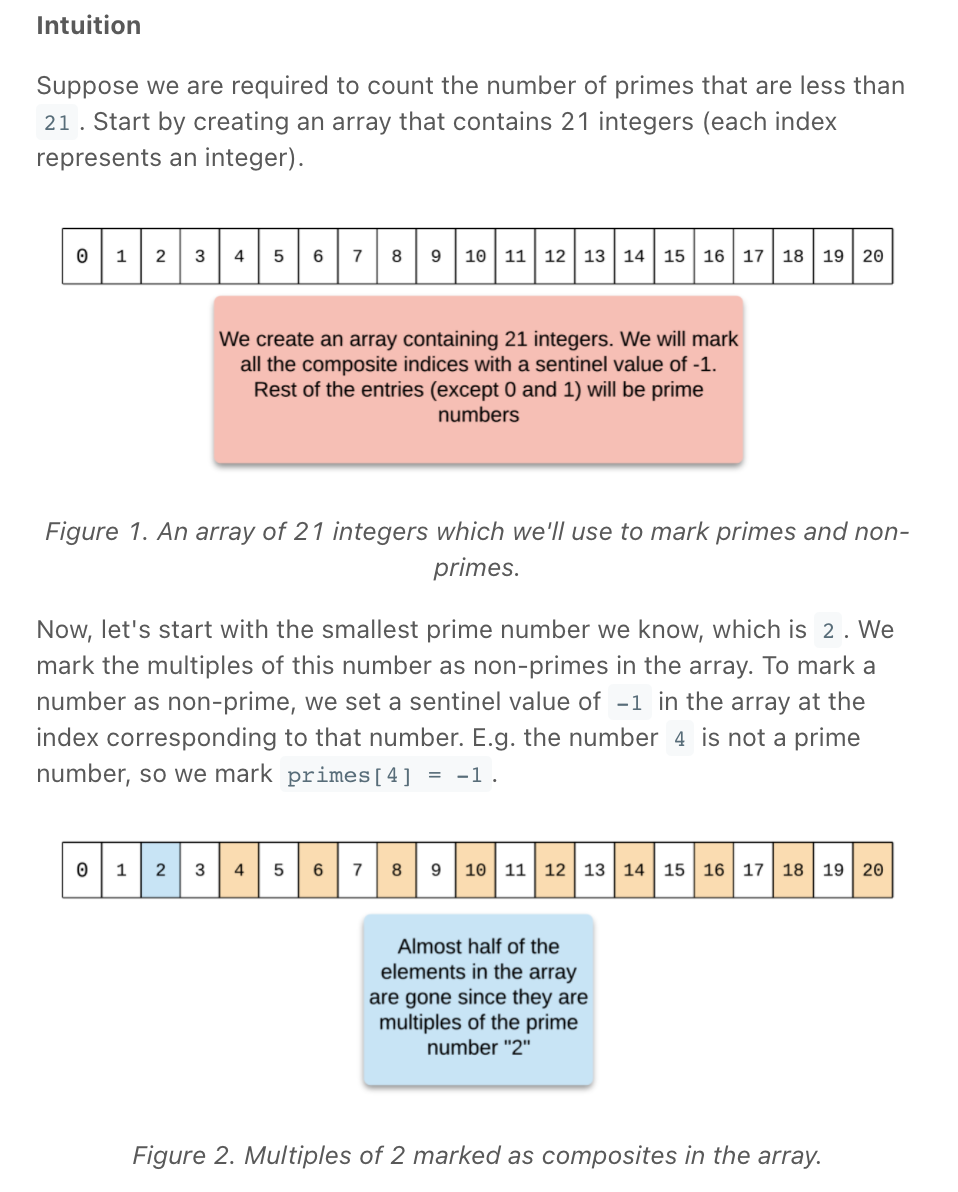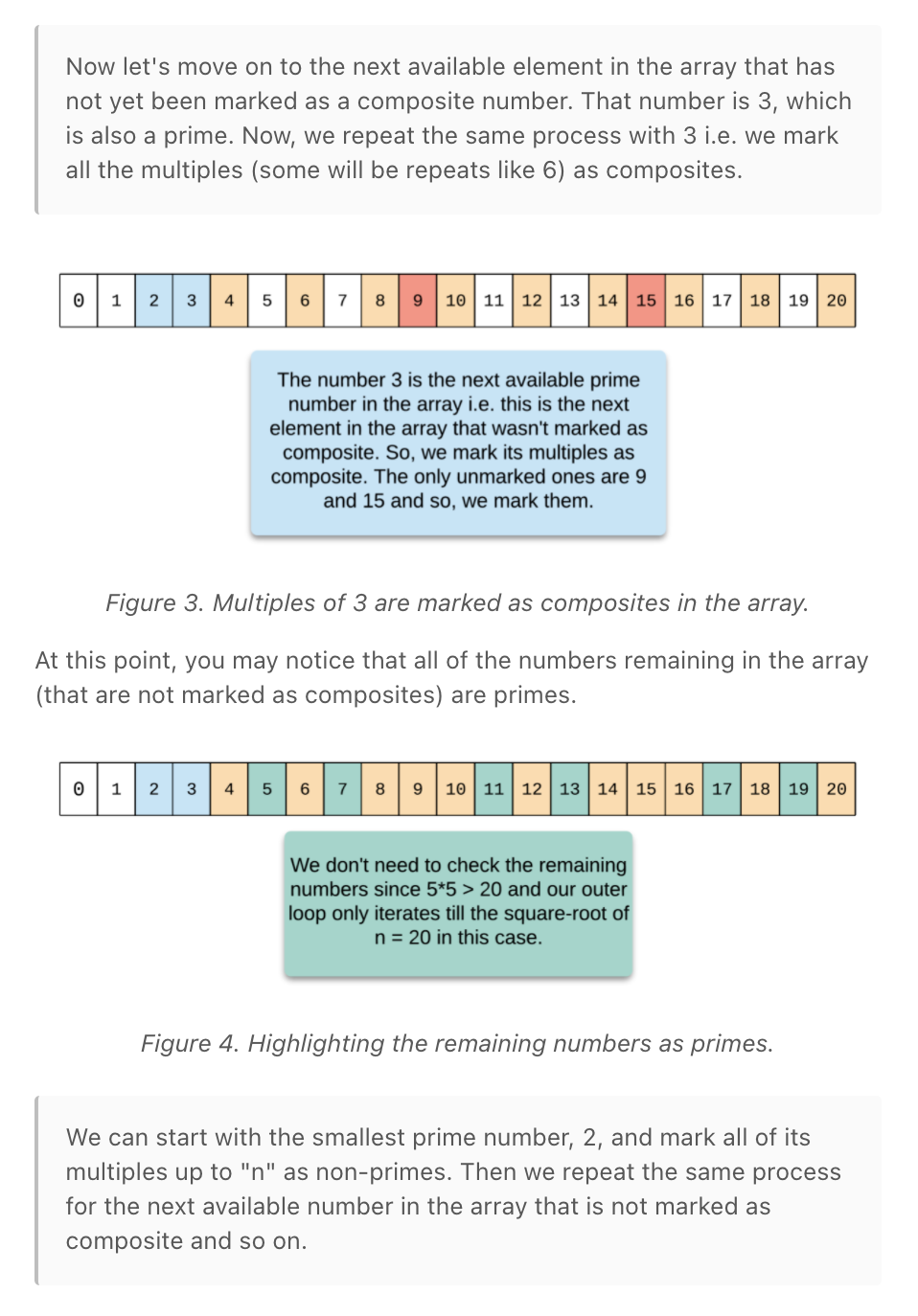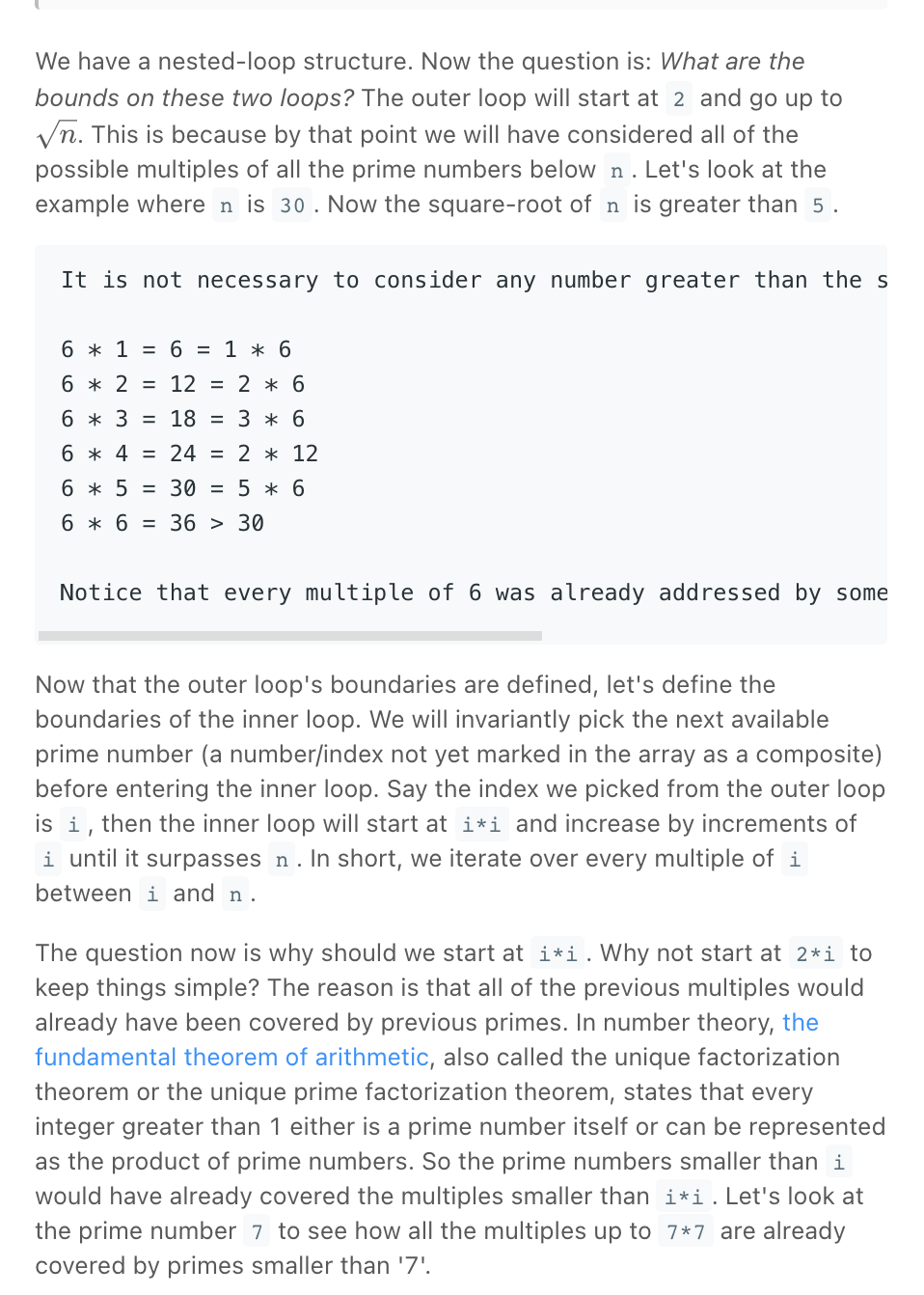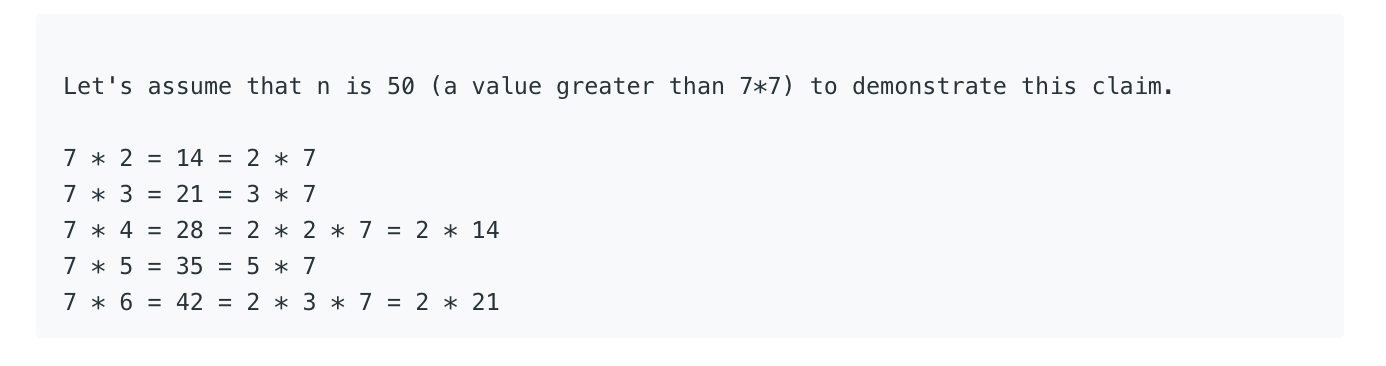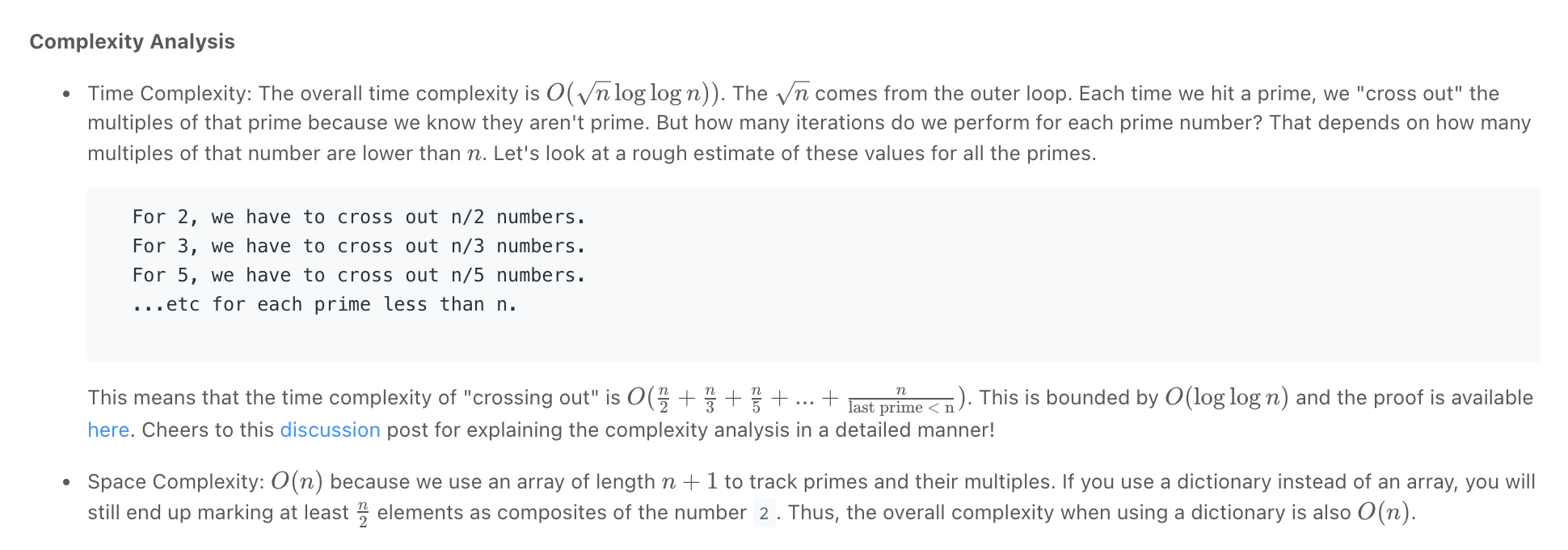"""
Count Primes:

Given an integer n, return the number of prime numbers that are strictly less than n.

Example 1:
Input: n = 10
Output: 4
Explanation: There are 4 prime numbers less than 10, they are 2, 3, 5, 7.
Example 2:
Input: n = 0
Output: 0
Example 3:
Input: n = 1
Output: 0

https://leetcode.com/problems/count-primes
"""

"""
The basic brute-force solution for this problem is to iterate from 0 to n and for each number, we do a prime-check.
To check if a number is prime or not, we simply check if its divisors include anything other than 1 and the number itself.
If so, then it is not a prime number. This method will not scale for the given limits on n.
The iteration itself has O(n) time complexity and for each iteration, we have the prime check which takes O(sqrt)O( n).
This will exceed the problem's time limit. Therefore, we need a more efficient solution.

Instead of checking if each number is prime or not, what if we mark the multiples of a prime number as non-prime?
"""

import math
class Solution_:  # times out on leetcode
def isPrime(self, n):

for num in range(2, math.floor(math.sqrt(n))):
if n % num == 0:
return False

return True

def countPrimes(self, n: int):
if n <= 2:
return 0

numbers = [-1] * n
numbers = False
numbers = False

for idx in range(2, n):
if numbers[idx] == False:
continue

numbers[idx] = self.isPrime(idx)

if numbers[idx]:  # only consider primes to ensure not calculating duplicates

multiplier = idx
while multiplier * idx < n:
numbers[multiplier * idx] = False
multiplier += 1

return numbers.count(True)

"""
"""

class Solution:
def countPrimes(self, n: int):
if n <= 2:
return 0

numbers = [True] * n
numbers = False
numbers = False

for idx in range(2, n):

if numbers[idx]:  # only consider primes to ensure not calculating duplicates

for multiple in range(idx+idx, n, idx):  # start,stop+1,step
numbers[multiple] = False

return numbers.count(True)

• Robot Bounded In Circle

"""
Robot Bounded In Circle:

On an infinite plane, a robot initially stands at (0, 0) and faces north.
The robot can receive one of three instructions:
"G": go straight 1 unit;
"L": turn 90 degrees to the left;
"R": turn 90 degrees to the right.
The robot performs the instructions given in order, and repeats them forever.
Return true if and only if there exists a circle in the plane such that the robot never leaves the circle.

Example 1:
Input: instructions = "GGLLGG"
Output: true
Explanation: The robot moves from (0,0) to (0,2), turns 180 degrees, and then returns to (0,0).
When repeating these instructions, the robot remains in the circle of radius 2 centered at the origin.
Example 2:
Input: instructions = "GG"
Output: false
Explanation: The robot moves north indefinitely.
Example 3:
Input: instructions = "GL"
Output: true
Explanation: The robot moves from (0, 0) -> (0, 1) -> (-1, 1) -> (-1, 0) -> (0, 0) -> ...

https://leetcode.com/problems/robot-bounded-in-circle
https://leetcode.com/problems/robot-bounded-in-circle/discuss/290856/JavaC%2B%2BPython-Let-Chopper-Help-Explain
"""
from enum import Enum
"""
MOVING:
- moving north is adding 1 to y
- moving left is adding 1 to x
- moving south is subreacting 1 from y
- moving left is subreacting 1 from x

DIRECTIONS:
north
west    east
south

SOLUTION:
- if we have a loop,
because the turnings are of 90 degrees,

if we are done with the instruction once and have changed direction by ((dir) cumulative direction which might be south, east, west)
it means that if this (dir) is applied at most 4 times (for east & west (horizontal)) or even 2 times (for north (vertical)) we will be back to the origin
"""

class Direction(Enum):
NORTH = "north"
SOUTH = "south"
EAST = "east"
WEST = "west"

class Solution:
def isRobotBounded(self, instructions: str):
position = [0, 0]
direction = Direction.NORTH

for _ in range(4):
# run instructions
for char in instructions:
if char == "G":
self.move(position, direction)
else:
direction = self.change_direction(direction, char)

# check if back to start
if position == 0 and position == 0:
return True

return False

def move(self, position, direction):
if direction == Direction.NORTH:
position = position+1
elif direction == Direction.SOUTH:
position = position-1
elif direction == Direction.EAST:
position = position+1
elif direction == Direction.WEST:
position = position-1

def change_direction(self, direction, change):
if change == "L":
if direction == Direction.NORTH:
return Direction.WEST
elif direction == Direction.SOUTH:
return Direction.EAST
elif direction == Direction.EAST:
return Direction.NORTH
elif direction == Direction.WEST:
return Direction.SOUTH
if change == "R":
if direction == Direction.NORTH:
return Direction.EAST
elif direction == Direction.SOUTH:
return Direction.WEST
elif direction == Direction.EAST:
return Direction.SOUTH
elif direction == Direction.WEST:
return Direction.NORTH

"""
---------------------------------------------
"""

class Solution1:
def isRobotBounded(self, instructions: str):
position = [0, 0]
direction = Direction.NORTH

for char in instructions:
if char == "G":
self.move(position, direction)
else:
direction = self.change_direction(direction, char)

# check if back to start
if position == 0 and position == 0:
return True
# check if changed direction
return direction != Direction.NORTH

def move(self, position, direction):
if direction == Direction.NORTH:
position = position+1
elif direction == Direction.SOUTH:
position = position-1
elif direction == Direction.EAST:
position = position+1
elif direction == Direction.WEST:
position = position-1

def change_direction(self, direction, change):
if change == "L":
if direction == Direction.NORTH:
return Direction.WEST
elif direction == Direction.SOUTH:
return Direction.EAST
elif direction == Direction.EAST:
return Direction.NORTH
elif direction == Direction.WEST:
return Direction.SOUTH
if change == "R":
if direction == Direction.NORTH:
return Direction.EAST
elif direction == Direction.SOUTH:
return Direction.WEST
elif direction == Direction.EAST:
return Direction.SOUTH
elif direction == Direction.WEST:
return Direction.NORTH

"""
---------------------------------------------
"""

# same as above but using math tricks
class Solution2:
def isRobotBounded(self, instructions: str):
direction = (0, 1)
start = [0, 0]

for x in instructions:
if x == 'G':
start += direction
start += direction
elif x == 'L':
direction = (-direction, direction)
elif x == 'R':
direction = (direction, -direction)

# check if back to start or if changed direction
return start == [0, 0] or direction != (0, 1)

• Integer to English Words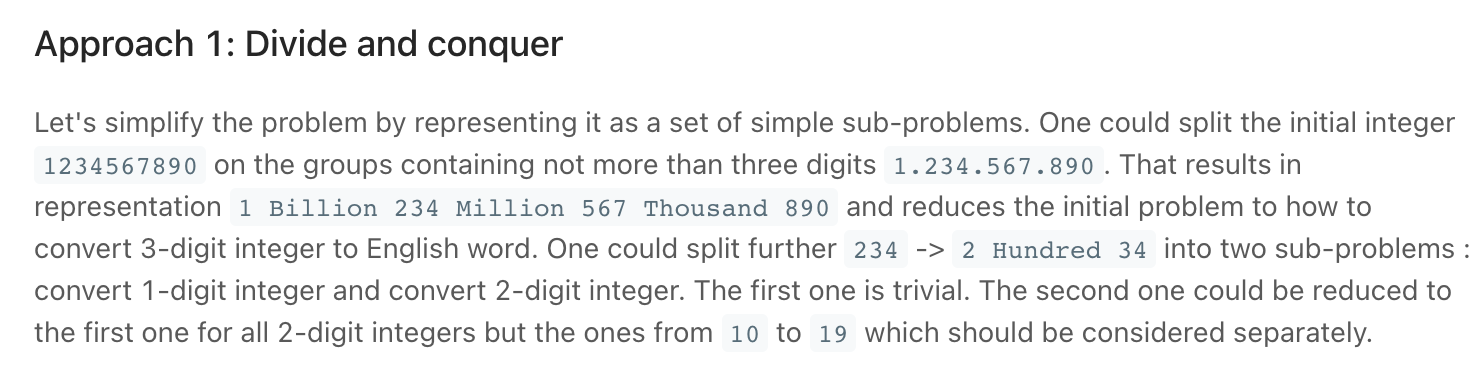Screen Recording 2021-10-15 at 21.00.14.mov

"""
Integer to English Words

Convert a non-negative integer num to its English words representation.

Example 1:
Input: num = 123
Output: "One Hundred Twenty Three"
Example 2:
Input: num = 12345
Output: "Twelve Thousand Three Hundred Forty Five"
Example 3:
Input: num = 1234567
Output: "One Million Two Hundred Thirty Four Thousand Five Hundred Sixty Seven"
Example 4:
Input: num = 1234567891
Output: "One Billion Two Hundred Thirty Four Million Five Hundred Sixty Seven Thousand Eight Hundred Ninety One"

https://leetcode.com/problems/integer-to-english-words
"""

"""
This problem is about the decomposition of the problem -- how do you break it down. Not about efficiency.

max => 2,147,483,647 ("Two Billion - One Hundred Forty Seven Million - Four Hundred Eighty Three Thousand - Six Hundred Forty Seven")

the len_three function and how it's used is what you should understand
"""

class Solution:

def ones(self, num):
store = {
1: 'One',
2: 'Two',
3: 'Three',
4: 'Four',
5: 'Five',
6: 'Six',
7: 'Seven',
8: 'Eight',
9: 'Nine'
}
return store[num]

def tens_less_20(self, num):
store = {
10: 'Ten',
11: 'Eleven',
12: 'Twelve',
13: 'Thirteen',
14: 'Fourteen',
15: 'Fifteen',
16: 'Sixteen',
17: 'Seventeen',
18: 'Eighteen',
19: 'Nineteen'
}
return store[num]

def tens_greater_20(self, num):
store = {
2: 'Twenty',
3: 'Thirty',
4: 'Forty',
5: 'Fifty',
6: 'Sixty',
7: 'Seventy',
8: 'Eighty',
9: 'Ninety'
}
return store[num]

def len_two(self, num):
if not num:
return ''
elif num < 10:
return self.ones(num)
elif num < 20:
return self.tens_less_20(num)
else:
tenner = num // 10
rest = num % 10

if rest:
return self.tens_greater_20(tenner) + ' ' + self.ones(rest)
else:
return self.tens_greater_20(tenner)

def len_three(self, num):
hundred = num // 100
rest = num % 100

if hundred and rest:
return self.ones(hundred) + ' Hundred ' + self.len_two(rest)
elif hundred and not rest:
return self.ones(hundred) + ' Hundred'
elif not hundred and rest:
return self.len_two(rest)

def numberToWords(self, num):

billion = num // 1000000000
million = (num - billion * 1000000000) // 1000000
thousand = (num - billion * 1000000000 - million * 1000000) // 1000
rest = num - billion * 1000000000 - million * 1000000 - thousand * 1000

if not num:
return 'Zero'

result = ''
if billion:
result = self.len_three(billion) + ' Billion'
if million:
result += ' ' if result else ''
result += self.len_three(million) + ' Million'
if thousand:
result += ' ' if result else ''
result += self.len_three(thousand) + ' Thousand'
if rest:
result += ' ' if result else ''
result += self.len_three(rest)
return result


Screen Recording 2021-10-15 at 21.29.59.mov

"""

Given two binary strings a and b, return their sum as a binary string.

Example 1:
Input: a = "11", b = "1"
Output: "100"
Example 2:
Input: a = "1010", b = "1011"
Output: "10101"

"""

class Solution:
def addBinary(self, a: str, b: str):
n = max(len(a), len(b))
res = *(n+1)

one_idx = len(a)-1
two_idx = len(b)-1
for idx in reversed(range(n+1)):
carry = res[idx]
one = 0
two = 0
if one_idx >= 0:
one = int(a[one_idx])
one_idx -= 1
if two_idx >= 0:
two = int(b[two_idx])
two_idx -= 1

addition = one + two + carry

res[idx] = addition % 2  # save result
# save carry
if addition // 2:  # prevent saving carry in idx 0

# remove preceeding zeros
start_idx = 0
while res[start_idx] == 0 and start_idx < len(res)-1:
start_idx += 1
res = res[start_idx:]

return "".join([str(item) for item in res])